# Graphing Calculator Drawing: Shading with Sine

This post is part of a series.

Demonstration - High Frequency. Observe the graph as you type each of the following inputs. In general, the graph of $y=\sin(vx)$ looks more and more solid as $v$ increases.

\begin{align*} y&=\sin(x) \\[10pt] y&=\sin(10x) \\[10pt] y&=\sin(100x) \\[10pt] y&=\sin(1000x) \end{align*}

Demonstration - Thickening a Curve. Observe the graph as you type each of the following inputs. In general, for large $v,$ the graph of $y=f(x)+A\sin(vx)$ thickens the curve $y=f(x)$ to have a vertical thickness of $2A.$

\begin{align*} y&=x+0.1\sin(1000x) \\[10pt] y&=x^2+0.1\sin(1000x) \\[10pt] y&=x^2+0.5\sin(1000x) \\[10pt] y&=\sin(x)+0.1\sin(1000x) \\[10pt] y&=\sin(x)+0.5\sin(1000x) \end{align*}

Demonstration - Varying Amplitude. Observe the graph as you type each of the following inputs. In general, when $v$ is large, the graph of $y=f(x)\sin(vx)$ shades the area between the graphs of $y=-f(x)$ and $y=f(x).$

\begin{align*} y&=x\sin(1000x) \\[10pt] y&=x^2\sin(1000x) \\[10pt] y&=\left( \sin x \right) \sin (1000x) \end{align*}

Demonstration - Shifts. Observe the graph as you type each of the following inputs. In general, the graph of $y=f(x-a)\sin(vx)+b$ is the graph of $y=f(x)\sin(vx)$ shifted right by $a$ units and up by $b$ units.

\begin{align*} y&=x^2 \sin(1000x) \\[10pt] y&=(x-2)\sin(1000x)+5 \end{align*}

Demonstration - Limitations. Observe the graph as you type each of the following inputs.

\begin{align*} y&=\sin(1000x) \lbrace 5 < x < 10 \rbrace \\[10pt] y&=(x-2)^2 \sin(1000x) \lbrace 2 < x < 4 \rbrace \\[10pt] y&=\left(\sqrt{x-3}\right)\sin(1000x)+5\left\{3<x<4\right\} \end{align*}

Exercise. Reproduce the downward parabola shown below.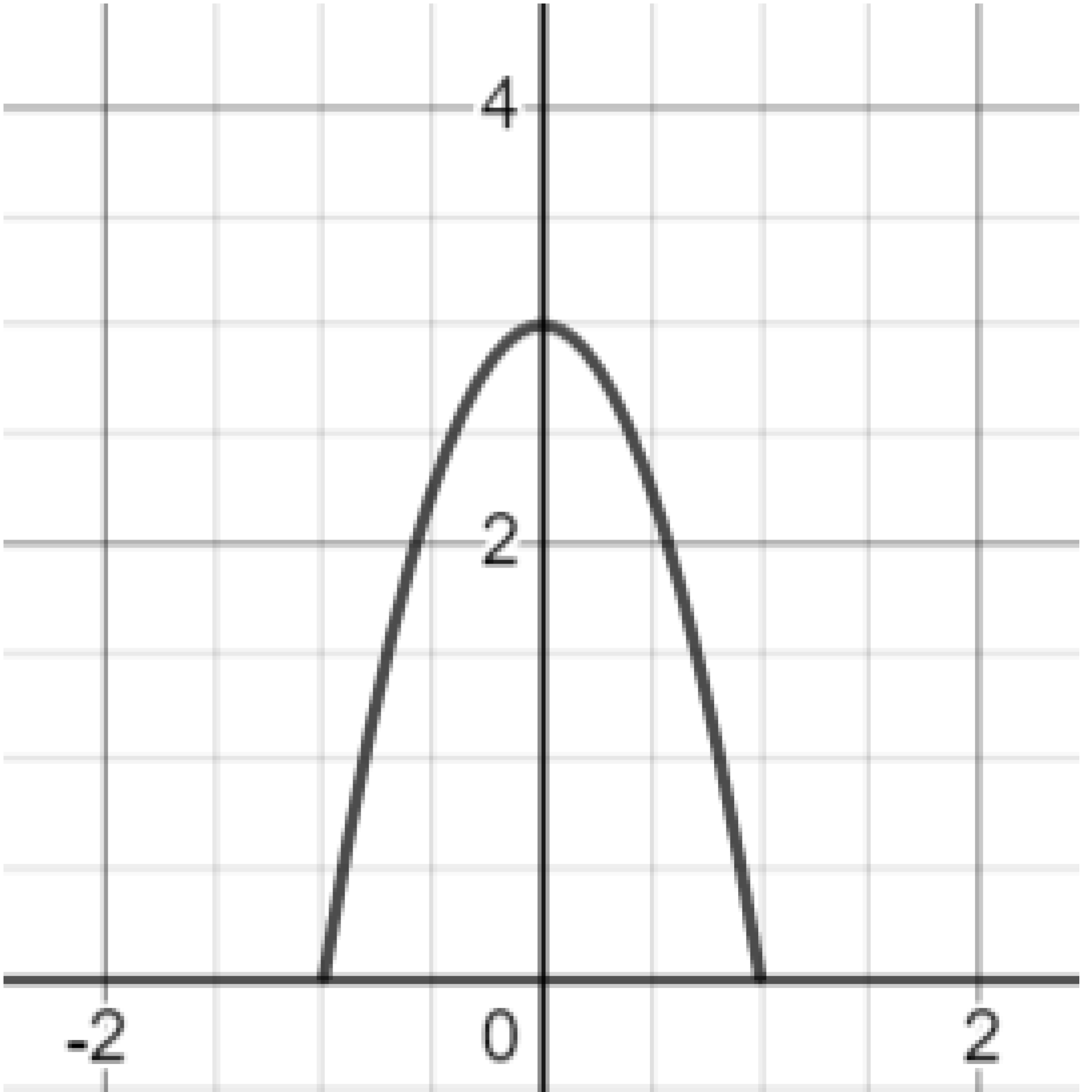Exercise. Use the parabola as the amplitude of a high-frequency sine function to create a shaded area.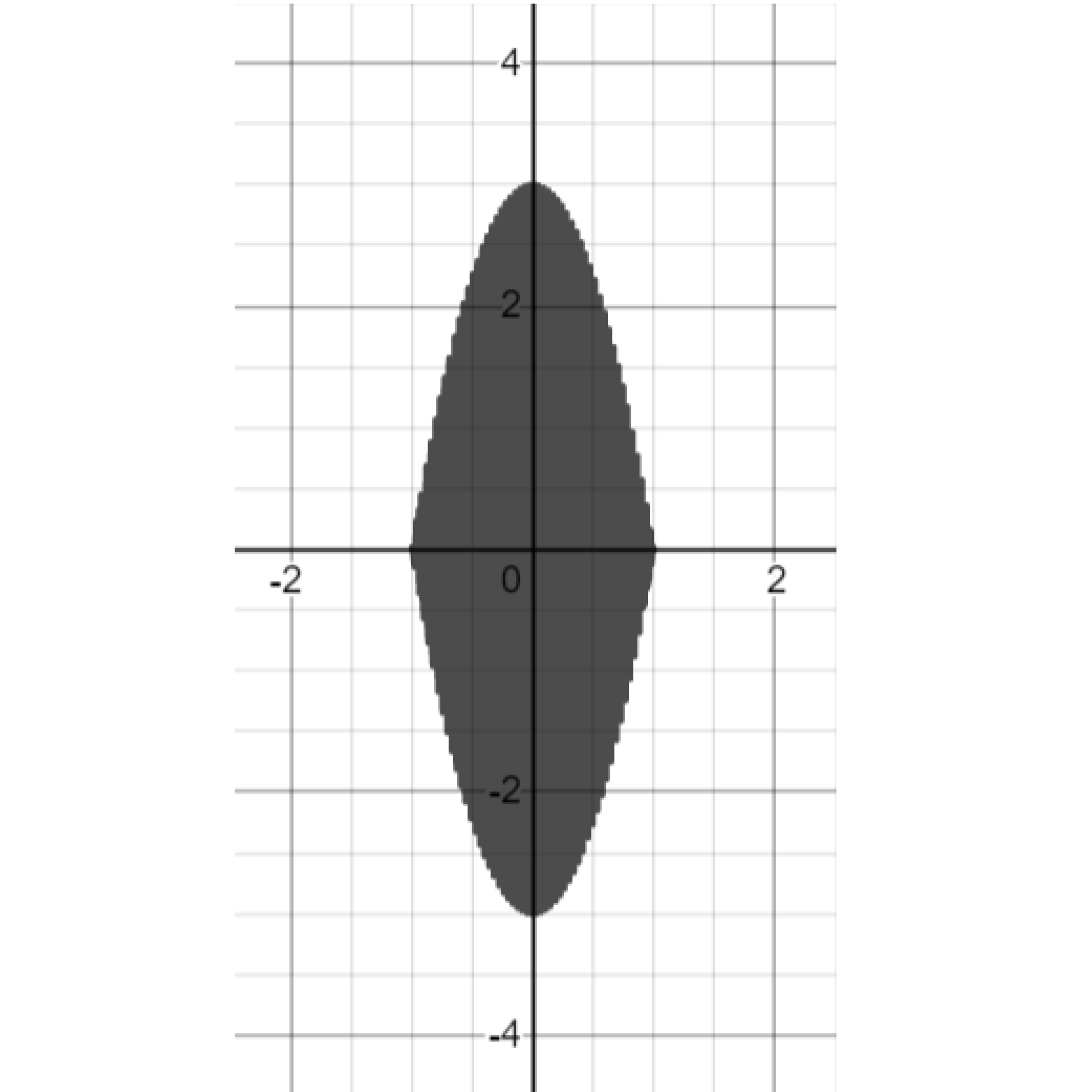Exercise. Shift the shaded area up and right.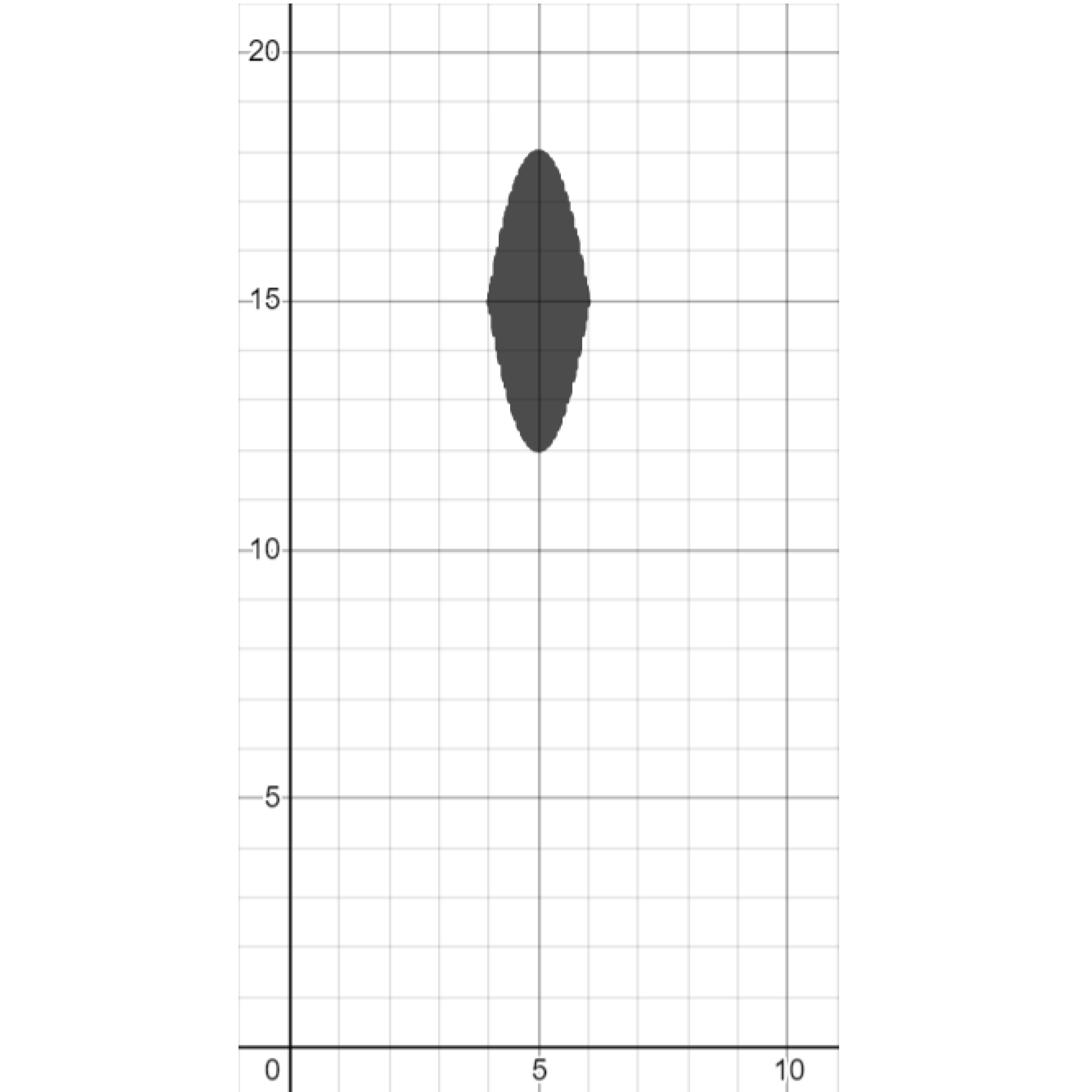Exercise. Draw parabolas around the shaded area to create an eye.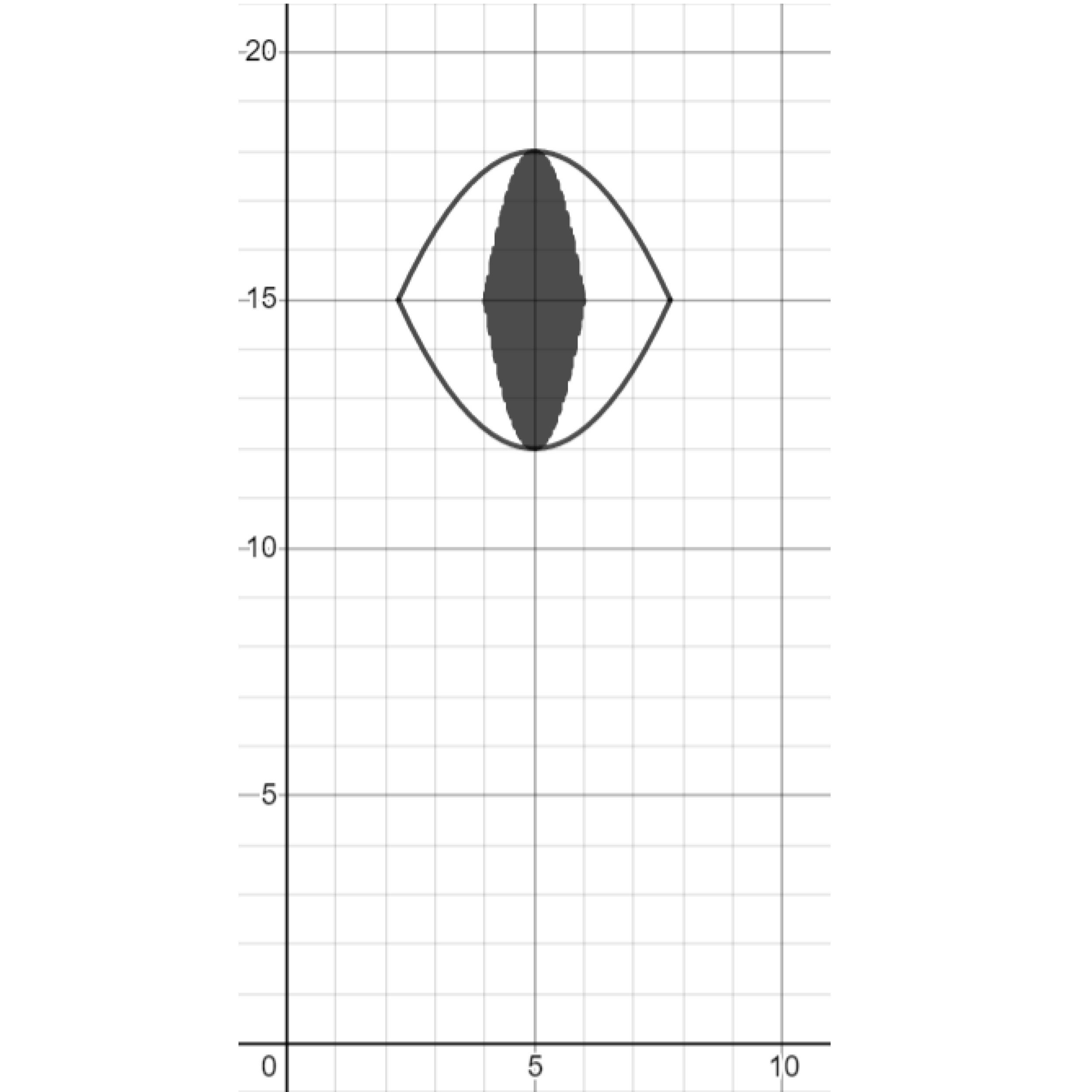Exercise. Create another eye.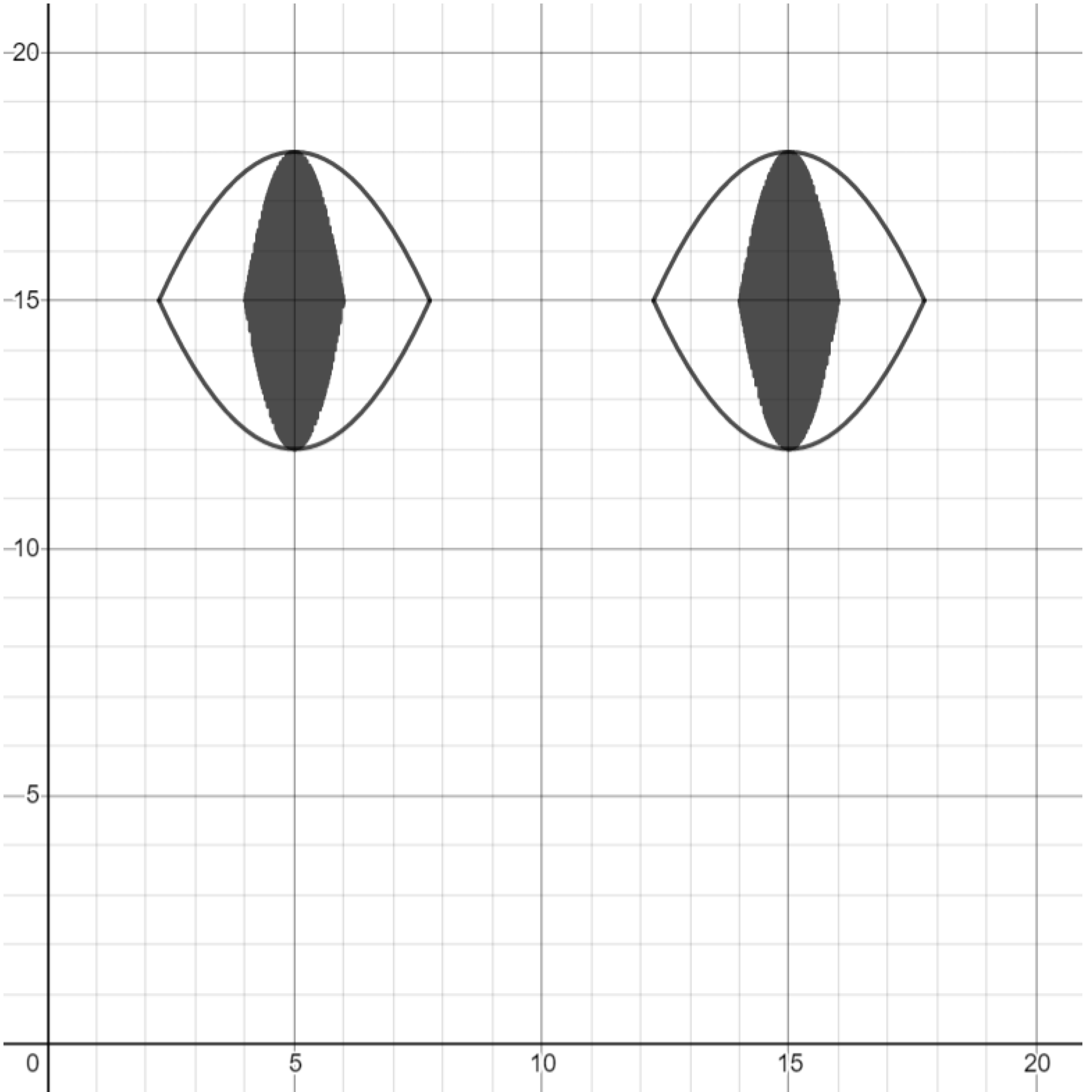Exercise. Create a parabola in the shape of a mouth.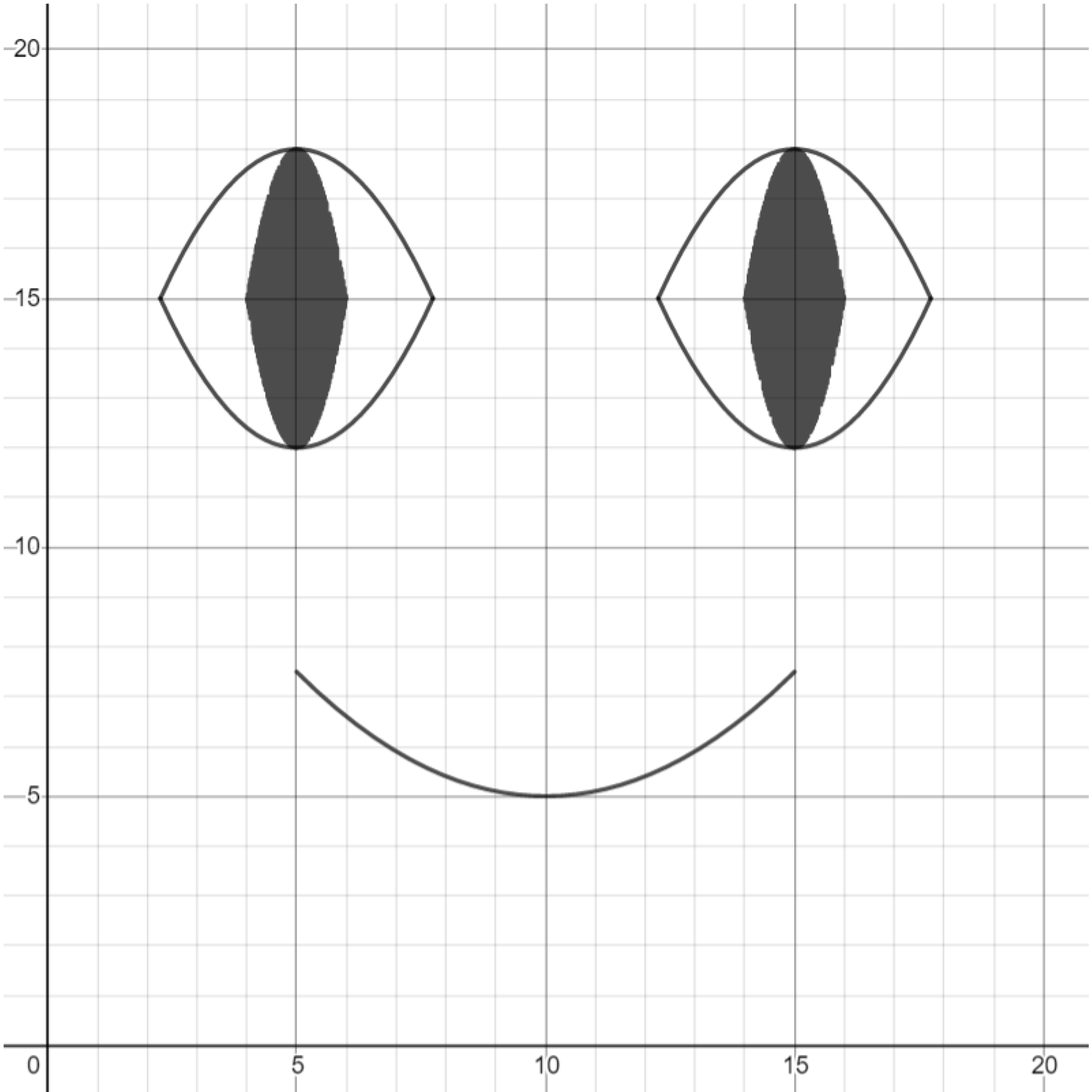Exercise. Thicken the parabola that forms the shape of the mouth.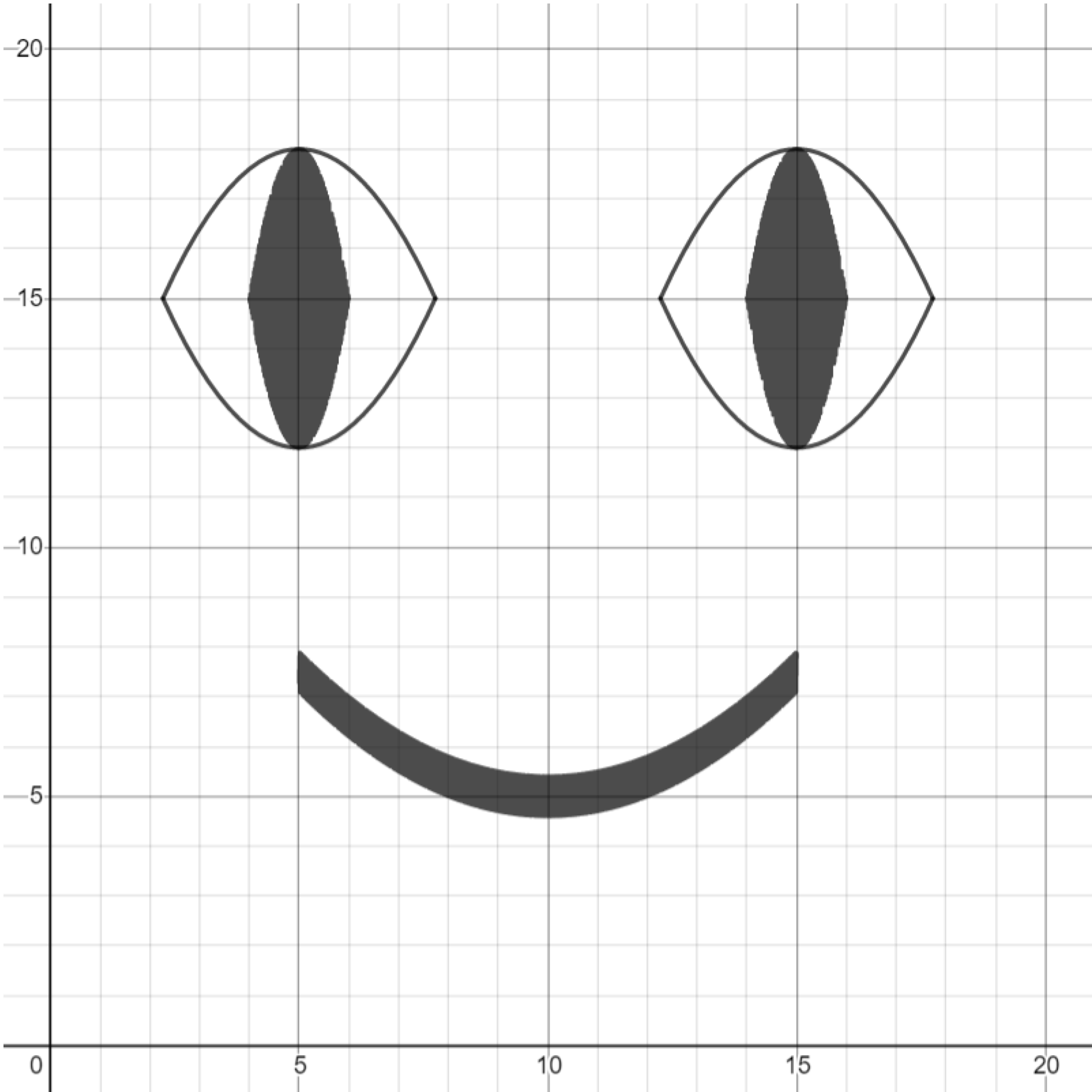Challenge. Make other kinds of emoji faces, such as a sad face or a laughing face.

This post is part of a series.

Tags: